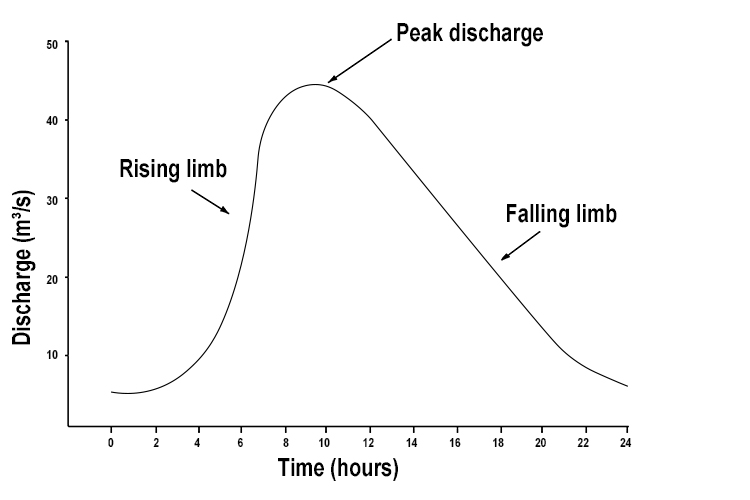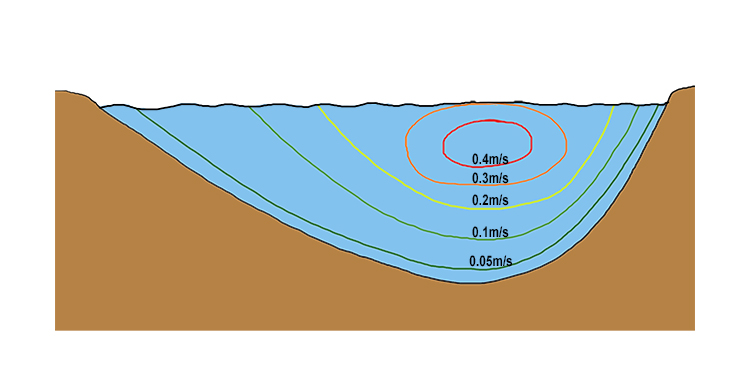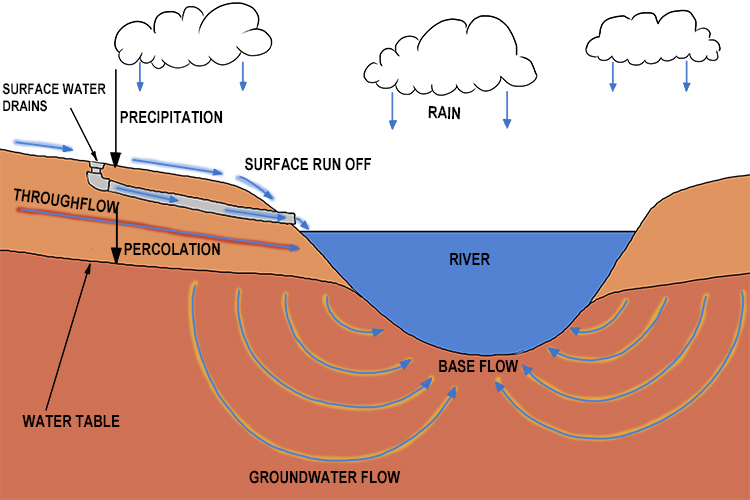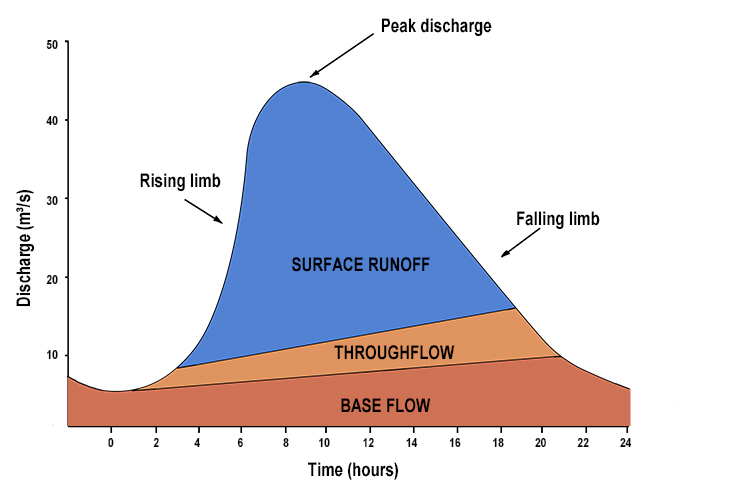# Discharge – The quantity of water that flows past a given point on a stream or river-bank within a given period of timeHe discharged (discharge) his gun as he stood at the start point and thousands (quantity) passed within minutes (time).

This helps to remind you that discharge is the quantity that passes a certain point in a certain time period.

The quantity of water that passes a point in a river is measured in cubic metres per second.The above is a typical plot of the average cubic metres of water passing a point in a river per second.

NOTE: We say the word average because if you look at the cross-section of a river the velocity of water passing this one point in the river varies:

Velocity of water per second through

a cut section of a riverSome clever calculations are made to work out how this translates to the average velocity or speed of the river per second.

The cubic metres of water per second is calculated by multiplying the cross-sectional area of the river by the average of the velocities shown above.

Cubic metre of water per second = Average velocity (m/s) x Area (m²)

The discharge of water at this point in the river is made up of several elements:This can be plotted on a graph as follows: